# Advanced Geometry : How to find the diagonal of a tetrahedron

## Example Questions

### Example Question #15 : Tetrahedrons

Given a regular tetrahedron with an edge of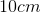, what is the height (or diagonal)? The height is the line drawn from one vertex perpendicular to the opposite face.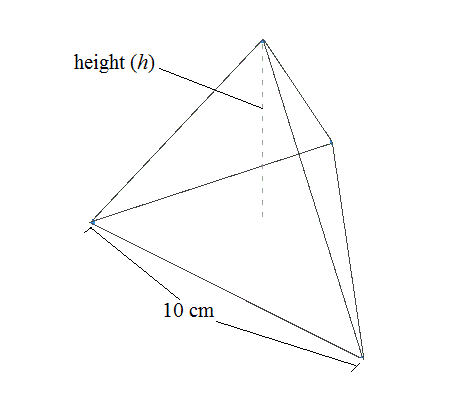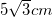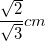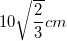None of the above.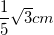Explanation:

The height of a regular tetrahedron can be derived from the formula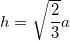whereis the length of one edge.

Therefore, plugging in the side length of,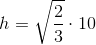.

### Example Question #16 : Tetrahedrons

Given a regular tetrahedron with an edge of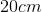, what is the height (or diagonal)? The height is the line drawn from one vertex perpendicular to the opposite face.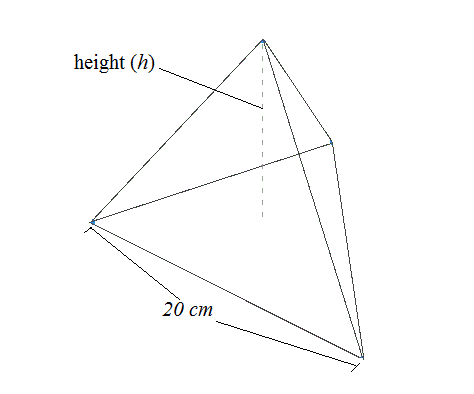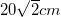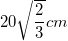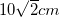None of the above.Explanation:

The height of a regular tetrahedron can be derived from the formulawhereis the length of one edge.

Plugging in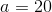we can solve for.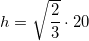### Example Question #17 : Tetrahedrons

Find the height of this regular tetrahedron: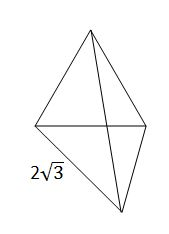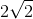Explanation:

The height of a regular tetrahedron can be found using the formula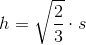where s is the length of the sides.

In this case, the sides have length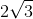, so we are multiplying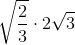.

We can simplify this by multiplying the numbers inside the radical: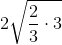, which simplifies to.